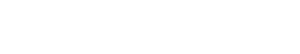# Quantile Range Outliers

Quantile Range Outliers (QR outliers) are defined as words that appear in a text document more than a certain number of standard deviations away from the mean. QR outliers are typically used to find unusual or unexpected terms in a document.

## How is Quantile Range Outliers Used Outside of Text Analytics?

The term “Quantile Range Outlier” can also be used more generally to refer to any value that falls outside of the range defined by the quantiles. For example, if you were looking at the ages of people in a room, and the first quartile was 20 years old, the second quartile was 30 years old, and the third quartile was 40 years old, then any person over 40 years old would be considered a Quantile Range Outlier.

## Quantile Range Outliers vs Quantile Deviation Outliers

It is important to note that Quantile Range Outliers should not be confused with Quantile Deviation Outliers. Quantile Deviation Outliers are defined as values that are a certain number of standard deviations away from the median, rather than the mean.

While both types of outliers can be used to find unusual values, they have different applications. For example, if you were looking at the heights of people in a room, and the first quartile was 5 feet tall, the second quartile was 6 feet tall, and the third quartile was 7 feet tall, then anyone 8 feet tall or taller would be considered a Quantile Deviation Outlier.

On the other hand, if you were looking at the ages of people in a room, and the first quartile was 20 years old, the second quartile was 30 years old, and the third quartile was 40 years old, then anyone over 40 years old would be considered a Quantile Range Outlier.

In general, Quantile Deviation Outliers are more useful for finding values that are far from the median, while Quantile Range Outliers are more useful for finding values that are far from the mean. It is also worth noting that there is no universally accepted definition of what constitutes an outlier. Different fields tend to use different cutoffs (for example, 3 standard deviations vs 2 standard deviations), and there is no one right answer.

The important thing is to be aware of the limitations of outliers, and to use them in conjunction with other methods (such as visual inspection) to get a complete picture.Unlock the power of actionable insights with AI-based natural language processing.